# 7.5 Decomposing Programs Into Communicating Processes

## 7.5 Decomposing Programs Into Communicating Processes

Not all problems can be divided into independent tasks. As we saw in Section 1.3.6, some applications are too large, in terms of their memory or compute needs, for a single processor or even a single SMP node. Solving these problems requires breaking the task into communicating (rather than independent) processes. In this section we will introduce two examples of decomposing a single task into multiple communicating processes. Because these programs are usually written using a message-passing programming model such as MPI or PVM, the details of implementing these examples are left to the chapters on these programming models.

### 7.5.1 Domain Decomposition

Many problems, such as the 3-dimensional partial differential equation (PDE) introduced in Section 1.3.6, are described by an approximation on a mesh of values. This mesh can be structured (also called regular) or unstructured. These meshes can be very large (as in the example in Chapter 1) and require more memory and computer power than a single processor or node can supply. Fortunately, the

For simplicity, we consider a two-dimensional example. A simple PDE is the Poisson equation,

 ∇2u = f in the interior, u = 0 on the boundary

where f is a given function and the problem is to find u. To further simplify the problem, we have chosen Dirichlet boundary conditions, which just means that the value of u along the boundary is zero. Finally, the domain is the unit square [0, 1] [0, 1]. A very simple discretization of this problem uses a finite difference approximation to the derivatives, yielding the approximation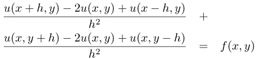Defining a mesh of points (xi, yj) = (i h, j h) with h = 1/n, and using the ui,j to represent the approximation of u(xi, yj), we get

 (7.1)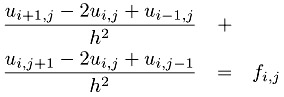We can now represent this using two dimensional arrays. We'll use Fortran because Fortran has some features that will make these examples easier to write. We will use U(i, j) as our computed value for ui,j.

To solve this approximation for the Poisson problem, we need to find the the values of U. This is harder than it may seem at first, because Equation 7.1 must be satisified at all points on the mesh (i.e., all values of i and j) simultaneously. In fact, this equation leads to a system of simultaneous linear equations. Excellent software exists to solve this problem (see Chapter 12), but we will use a very simple approach to illustrate how this problem can be parallelized. The first step is to write this problem as an iterative process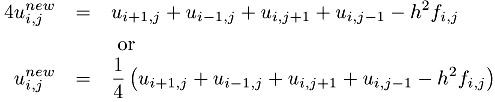This is the Jacobi iteration, and can be written in Fortran as

`    real UNEW(0:n,0:n), U(0:n,0:n), F(1:n-1,1:n-1)    ... code to initialize U and F    do iter=1,itermax        do j=1,n-1            do i=1,n-1                UNEW(i,j) = 0.25 * (U(i+1,j)+U(i-1,j) +     &                                    U(i,j+1)+U(i,j-1) - F(i,j))            enddo        enddo        ... code to determine if the iteration has converged    enddo `

At this point, we can see how to divide this problem across multiple processors. The simplest approach is to divide the mesh into small pieces, giving each piece to a separate processor. For example, we could divide the original mesh (U(0:n,0:n) in the code) into two parts: U(0:n,0:n/2) and (U(0:n,n/2+1:n). This approach is called domain decomposition, and is based on using the decompositions of the physical domain (the unit square in this case) to create parallelism.

Applying this approach for two processors, we have the two code fragments shown in Figure 7.12. Note that each process now has only half of the data because each array is declared with only the data "owned" by that processor. This also shows why we used Fortran; the ability to specify the range of the indices for the arrays in Fortran makes these codes very easy to write.Code for process zero

`    real UNEW(0:n,0:n/2), U(0:n,0:n/2), F(1:n-1,1:n/2)    ... code to initialize u and f    do iter=1,itermax        do j=1,n/2            do i=1,n-1                UNEW(i,j) = 0.25 * (U(i+1,j)+U(i-1,j) +     &                                    U(i,j+1)+U(i,j-1) - F(i,j))            enddo        enddo        ... code to determine if the iteration has converged    enddo `

Code for process one

`    real UNEW(0:n,n/2+1:n), U(0:n,n/2+1:n), F(1:n-1,n/2+1:n-1)    ... code to initialize u and f    do iter=1,itermax        do j=n/2+1,n-1            do i=1,n-1                UNEW(i,j) = 0.25 * (U(i+1,j)+U(i-1,j) +     &                                    U(i,j+1)+U(i,j-1) - F(i,j))            enddo        enddo        ... code to determine if the iteration has converged    enddo `Figure 7.12: Two code fragments for parallelizing the Poisson problem with the Jacobi iteration

However, unlike the decompositions into independent tasks in the first part of this chapter, this decomposition does not produce indepentent tasks. Consider the case of j=n/2 in the original code. Process zero in Figure 7.12 computes the values of UNEW(i,n/2). However, to do this, it needs the values of U(i,n/2+1). This data is owned by processor one. In order to make this code work, we must communicate the data owned by processor one (the values of U(i,n/2+1) for i=1,...,n-1) to processor zero. We must also allocate another row of storage to hold these values; this extra row is often called a ghost points or a halo. The resulting code is shown in Figure 7.13.Code for process zero

`    real UNEW(0:n,0:n/2+1), U(0:n,0:n/2+1), F(1:n-1,1:n/2)    ... code to initialize u and f    do iter=1,itermax        ... code to Get u(i,n/2+1) from process one        do j=1,n/2            do i=1,n-1                UNEW(i,j) = 0.25 * (U(i+1,j)+U(i-1,j) +     &                                    U(i,j+1)+U(i,j-1) - F(i,j))            enddo        enddo        ... code to determine if the iteration has converged    enddo `

Code for process one

`    real UNEW(0:n,n/2:n), U(0:n,n/2:n), F(1:n-1,n/2+1:n-1)    ... code to initialize u and f    do iter=1,itermax        ... code to Get u(i,n/2) from process zero        do j=n/2+1,n-1            do i=1,n-1                UNEW(i,j) = 0.25 * (U(i+1,j)+U(i-1,j) +     &                                    U(i,j+1)+U(i,j-1) - F(i,j))            enddo        enddo        ... code to determine if the iteration has converged    enddo `Figure 7.13: Two code fragments for parallelizing the Poisson problem with the Jacobi iteration, including the communication of ghost points. Note the changes in the declarations for U and UNEW.

Note also that although both processes have variables named UNEW and i, these are different variables. This kind of parallel programming model is sometimes called a shared-nothing model because no data (variables or instructions) are shared between the processes. Instead, explicit communication is required to move data from one process to another. Section 8.3 discusses this example in detail, using the Message Passing Interface (MPI) to implement the communication of data between the processors, using code written in C.

There are more complex and powerful domain decomposition techniques, but they all start from dividing the domain (usually the physical domain of the problem) into a number of separate pieces. These pieces must communicate along their edges at each step of the computation. As described in Section 1.3.6, a decomposition into squares (in two-dimensions) or cubes (in three dimensions) reduces the amount of data that must be communicated because those shapes maximize the volume to surface area ratio for rectangular solids.

### 7.5.2 Data Structure Decomposition

Not all problems have an obvious decomposition in terms of a physical domain. For these problems, a related approach that decomposes the data-structures in the application can be applied. An example of this kind of application is the solution of a system of linear equations Ax = b, where the equations are said to be dense. This simply means that most of the elements of the matrix describing the problem are non-zero. A good algorihm for solving this problem is called LU factorization, because it involves first computing a lower trianular matrix L and an upper triangular matrix U such that the original matrix A is given by the product LU. Because an lower (resp. upper) triangular matrix has only zero elements below (resp. above) the diagonal, it is easy to find the solution x once L and U are known. This is the algorithm used in the LINPACK  benchmark. A parallel verison of this is used in the High-Performance Linpack benchmark, and this section will sketch out some of the steps used in parallelizing this kind of problem.

The LU factorization algorithm looks something like the code shown in Figure 7.14, an n n matrix A represented by the Fortran array a(n,n).` real a(n,n) do i=i, n    do k=1,i-1        sum = 0        do j=1,k-1            sum = sum + a(i,j)*a(j,k)        enddo        a(i,k) = (a(i,k) - sum) / a(k,k)    enddo    do k=1,i        sum = 0        do j=1,k-1            sum = sum + a(k,j)*a(j,i)        enddo        a(k,i) = a(k,i) - sum    enddo enddo `Figure 7.14: LU Factorization code. The factors L and U are computed in-place; that is, they are stored over the input matrix a.

An obvious way to decompose this problem, following the domain decomposition discussion, is to divide the matrix into groups of rows (or groups of columns):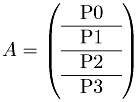However, this will yield an inefficient program. Because of the outer-loop over the rows of the matrix (the loop over i), once i reaches n/4 in the case of four processors, processor zero has no work left to do. As the computation proceeds, fewer and fewer processors can help with the computation. For this reason, more complex decompositions are used. For example, the ScaLAPACK library uses the two-dimensional block-cyclic distribution shown here: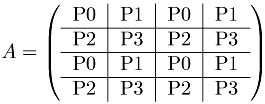This decomposition ensures that most processors are in use until the very end of the algorithm.

Just as in the domain decomposition example, communication is required to move data from one processor to another. In this example, data from the ith row must be communicated from the processors that hold that data to the processors holding the data needed for the computations (the loops over j). We do not show the communication here; see the literature on solving dense linear systems in parallel for details on these algorithms.

The technique of dividing the data structure among processors is a general one. Chosing the decomposition to use requires balancing the issues of load balance, communication, and algorithm complexity. Addressing these may suggest algorithmic modifications to provide better parallel performance. For example, certain variations of the LU factorization method described above may perform the floating-point operations in a different order. Because floating-point arithmetic is not associative, small differences in the results may occur. Other variations may produce answers that are equally valid as approximations but give results that are slightly different. Care must be exercised here, however, because some approximations are better behaved than others. Before changing the algorithm, make sure that you understand the consequences of any change. Consult with a numerical analysist or read about stability and well-posedness in any textbook on numerical computing.

### 7.5.3 Other Approaches

There are many techniques for creating parallel algorithms. Most involve dividing the problem into separate tasks that may need to communicate. For an effective decomposition for a Beowulf cluster, the amount of computation must be large relative to the amount of communication. Examples of these kinds of problems include sophisticated search and planning algorithms, where the results of some tests are used to speed up other tests (for example, a computation may discover that a subproblem has already been solved.).

Some computations are implemented as master/worker applications, where each worker is itself a parallel program (e.g., because of the memory needs or the requirement that the computation finish within a certain amount of time, such as overnight). Master/worker algorithms can also be made more sophisticated, guiding the choice and order of worker tasks by previous results returned by the workers.Beowulf Cluster Computing With Linux 2003
ISBN: N/A
EAN: N/A
Year: 2005
Pages: 198